Foxtable(狐表)用户栏目专家坐堂 → 会计凭证的设计和录入

共有850人关注过本帖树形打印复制链接

# 主题：会计凭证的设计和录入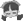31楼 | 信息 | 搜索 | 邮箱 | 主页 | UC加好友发短信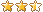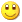Post By：2021/4/6 17:35:00 [只看该作者]

 Case "收款凭证_Table1"            Select Case e.Node.Level                Case 0                    tr("贷方科目") = Vals(0)                    e.Form.DropDownBox.Value = tr("贷方科目")                    e.Form.DropDownBox.CloseDropdown()                Case 1                    tr("贷方科目") = Vals(0)                    tr("明细科目") = Vals(1).SubString(8,Vals(1).Length - 8)                    e.Form.DropDownBox.Value = tr("贷方科目")                    e.Form.DropDownBox.CloseDropdown()                Case 2                    tr("贷方科目") = Vals(0)                    tr("明细科目") = Vals(1).SubString(8,Vals(1).Length - 8) & "-" & Vals(2).SubString(12,Vals(2).Length - 12)                    e.Form.DropDownBox.Value = tr("贷方科目")                    e.Form.DropDownBox.CloseDropdown()                Case 3                    tr("贷方科目") = Vals(0)                    tr("明细科目") = Vals(1).SubString(8,Vals(1).Length - 8) & "-" & Vals(2).SubString(12,Vals(2).Length - 12) & "-" & Vals(3).SubString(16,Vals(3).Length - 16)                    e.Form.DropDownBox.Value = tr("贷方科目")                    e.Form.DropDownBox.CloseDropdown()                Case 4                    tr("贷方科目") = Vals(0)                    tr("明细科目") = Vals(1).SubString(8,Vals(1).Length - 8) & "-" & Vals(2).SubString(12,Vals(2).Length - 12) & "-" & Vals(3).SubString(16,Vals(3).Length - 16) & "-" & Vals(4).SubString(20,Vals(4).Length - 20)                    e.Form.DropDownBox.Value = tr("贷方科目")                    e.Form.DropDownBox.CloseDropdown()            End Select        Case "付款凭证_Table1"            Select Case e.Node.Level                Case 0                    tr("借方科目") = Vals(0)                    e.Form.DropDownBox.Value = tr("借方科目")                    e.Form.DropDownBox.CloseDropdown()                Case 1                    tr("借方科目") = Vals(0)                    tr("明细科目") = Vals(1).SubString(8,Vals(1).Length - 8)                    e.Form.DropDownBox.Value = tr("借方科目")                    e.Form.DropDownBox.CloseDropdown()                Case 2                    tr("借方科目")= Vals(0)                    tr("明细科目") = Vals(1).SubString(8,Vals(1).Length - 8) & "-" & Vals(2).SubString(12,Vals(2).Length - 12)                    e.Form.DropDownBox.Value = tr("借方科目")                    e.Form.DropDownBox.CloseDropdown()                Case 3                    tr("借方科目") = Vals(0)                    tr("明细科目") = Vals(1).SubString(8,Vals(1).Length - 8) & "-" & Vals(2).SubString(12,Vals(2).Length - 12) & "-" & Vals(3).SubString(16,Vals(3).Length - 16)                    e.Form.DropDownBox.Value = tr("借方科目")                    e.Form.DropDownBox.CloseDropdown()                Case 4                    tr("借方科目") = Vals(0)                    tr("明细科目") = Vals(1).SubString(8,Vals(1).Length - 8) & "-" & Vals(2).SubString(12,Vals(2).Length - 12) & "-" & Vals(3).SubString(16,Vals(3).Length - 16) & "-" & Vals(4).SubString(20,Vals(4).Length - 20)                    e.Form.DropDownBox.Value = tr("借方科目")                    e.Form.DropDownBox.CloseDropdown()            End Select    End SelectEnd If项目看28楼上传的文件。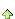32楼 | 信息 | 搜索 | 邮箱 | 主页 | UC加好友发短信Post By：2021/4/7 11:24:00 [只看该作者]

这是未改前备份，记账凭证窗口中的表绑定记账凭证表，会计科目以目录树输入没问题，因为窗口中的数据修改后再保存，如果窗口中的表不是绑定后台数据源表，就会出现重复保存问题，再三追问只能以绑定后台数据源表解决，所以我只好把记账凭证窗口中原绑定记账凭证表的表改为绑定财务数据源表，这一改动，记账凭证窗口中的会计科目自动录入就出问题了，查了很久不知怎么解决。
 下载信息  [文件大小：   下载次数： ]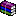点击浏览该文件:小区车辆管理（保存）.rar

[此贴子已经被作者于2021/4/7 13:18:11编辑过]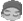33楼 | 信息 | 搜索 | 邮箱 | 主页 | UC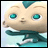加好友发短信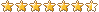Post By：2021/4/7 14:23:00 [只看该作者]

 下载信息  [文件大小：   下载次数： ]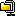点击浏览该文件:小区车辆管理（0406）.zip34楼 | 信息 | 搜索 | 邮箱 | 主页 | UC加好友发短信Post By：2021/4/7 15:39:00 [只看该作者]

 下载信息  [文件大小：   下载次数： ]点击浏览该文件:小区车辆管理（0407）.rar35楼 | 信息 | 搜索 | 邮箱 | 主页 | UC加好友发短信Post By：2021/4/7 16:03:00 [只看该作者]

 去掉窗口表DataColChanging事件36楼 | 信息 | 搜索 | 邮箱 | 主页 | UC加好友发短信Post By：2021/4/8 14:44:00 [只看该作者]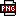此主题相关图片如下：qq图片20210408143358.png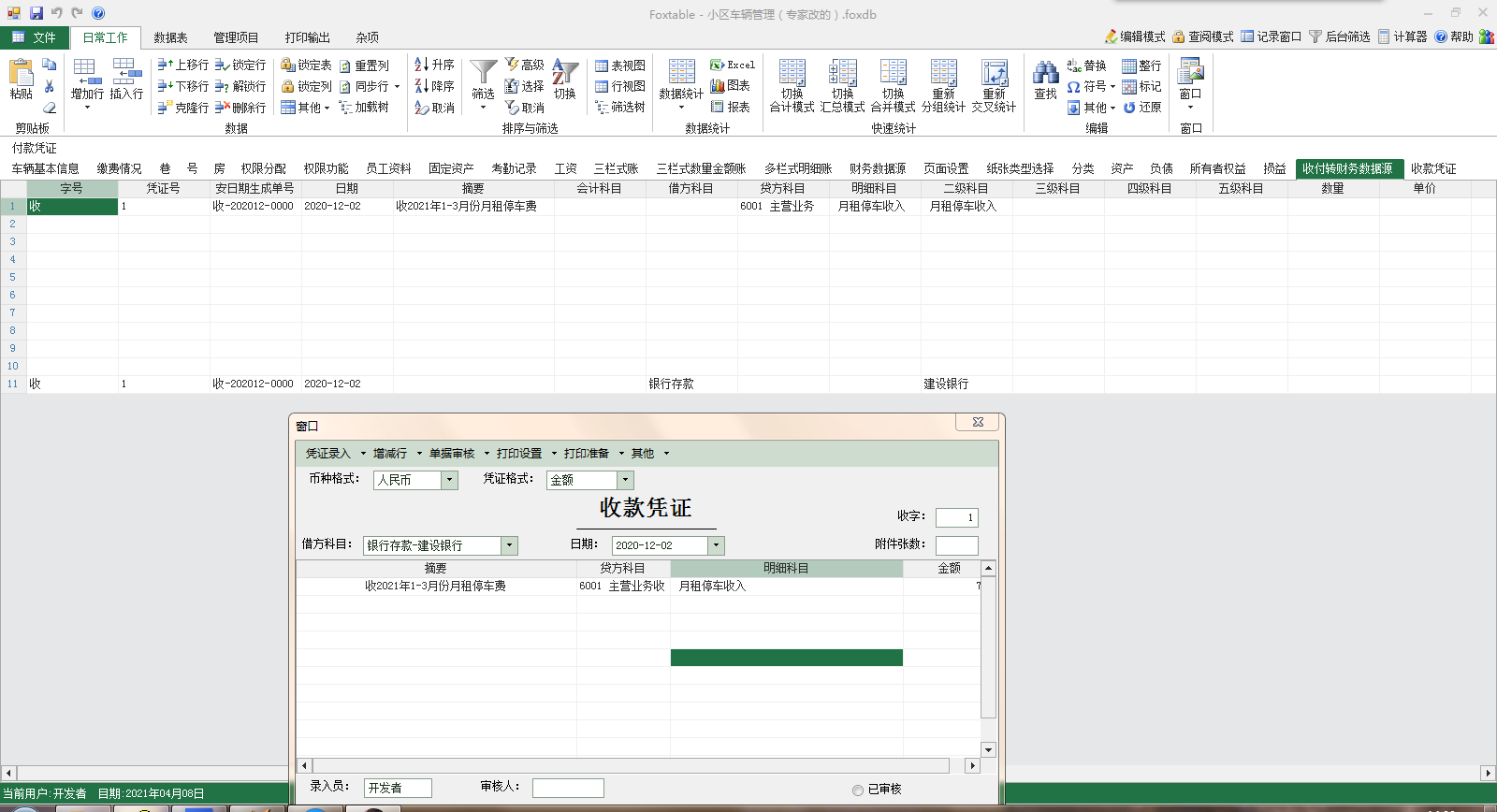'一.数据录入:Select Case e.StripItem.Name    Case "保存" '保存代        If e.Form.Controls("rq").text = "" Then            messagebox.show("请选择日期")            Return        ElseIf Tables("收款凭证_Table1").Compute("Count([贷方科目])") = 0 Then            Return        Else            Dim r As DataRow = DataTables("收付转财务数据源").SQLFind("[字号] = '收' And Month([日期]) = " & Month(e.Form.Controls("rq").Value) & " And [凭证号] = '" & e.Form.Controls("dh").text & "'")            If r Is Nothing Then                r = DataTables("收付转财务数据源").addnew                r("日期") = e.Form.Controls("rq").text                r("凭证号") = e.Form.Controls("dh").text                r("安日期生成单号辅助列") = e.Form.Controls("dh1").text                r("附件张数") = e.Form.Controls("TextBox1").text                r("录入员") = e.Form.Controls("lry").text                r("字号") = "收"                r("操作人姓名") = User.Name & " " & Date.Now                r("借或贷") = "借"                Dim Tel0 As String = e.Form.Controls("ComboBox3").text                Dim Parts0() As String = Tel0.Split("-")                If Parts0.length > 0 Then                    r("借方科目") = Parts0(0)                    If Parts0.length > 1 Then                        r("二级科目") = Parts0(1)                    End If                End If                r("借方金额") = Tables("收款凭证_Table1").DataTable.Compute("Sum(贷方金额)")            ElseIf r IsNot Nothing And r("借或贷") = "借" Then                r("日期") = e.Form.Controls("rq").text                r("附件张数") = e.Form.Controls("TextBox1").text                r("录入员") = e.Form.Controls("lry").text                r("操作人姓名") = User.Name & " " & Date.Now                Dim Tel0 As String = e.Form.Controls("ComboBox3").text                Dim Parts0() As String = Tel0.Split("-")                If Parts0.length > 0 Then                    r("借方科目") = Parts0(0)                    If Parts0.length > 1 Then                        r("二级科目") = Parts0(1)                    End If                End If                r("借方金额") = Tables("收款凭证_Table1").DataTable.Compute("Sum(贷方金额)")            End If            DataTables("收付转财务数据源").Save()            For Each dt As DataRow In Tables("收款凭证_Table1").DataTable.Select("[贷方科目] Is not null")                Dim Tel As String = dt("明细科目")                Dim Parts() As String = Tel.Split("-")                dt("日期") = e.Form.Controls("rq").text                dt("凭证号") = e.Form.Controls("dh").text                dt("安日期生成单号辅助列") = e.Form.Controls("dh1").text                dt("附件张数") = e.Form.Controls("TextBox1").text                dt("录入员") = e.Form.Controls("lry").text                dt("字号") = "收"                dt("借或贷") = "贷"                dt("操作人姓名") = User.Name & " " & Date.Now                If Parts.length > 0 Then                    dt("二级科目") = Parts(0)                    If Parts.length > 1 Then                        dt("三级科目") = Parts(1)                        If Parts.length > 2 Then                            dt("四级科目") = Parts(2)                            If Parts.length > 3 Then                                dt("五级科目") = Parts(3)                                dt.Save                            End If                        End If                    End If                End If            Next        End IfEnd Select　　上面的代码红色标注的借方代码有问题，没得出借方金额，它应等于贷方金额的合计，我无论直接引用贷方合计金额，还是统计贷方金额都没有结果，还的借方数据的行要在贷方数据的行上，这里走到贷方数据下了，另保存时未关闭收款凭证窗口前都见中间很多空行，我要在收付转财务数据源表中不产生这些空行。　 [此贴子已经被作者于2021/4/8 14:46:19编辑过]37楼 | 信息 | 搜索 | 邮箱 | 主页 | UC加好友发短信Post By：2021/4/8 14:50:00 [只看该作者]

 其实这样写一旦改凭证号码借方会重复添加一行，原来的行还在。38楼 | 信息 | 搜索 | 邮箱 | 主页 | UC加好友发短信Post By：2021/4/8 15:33:00 [只看该作者]

 改为sqltable直接加载编辑。当然您喜欢钻牛角尖我也没有办法。39楼 | 信息 | 搜索 | 邮箱 | 主页 | UC加好友发短信Post By：2021/4/8 15:43:00 [只看该作者]

 表是SQLTable表，贷方是没问题的，借方在控件上，只能这样录入啊。40楼 | 信息 | 搜索 | 邮箱 | 主页 | UC加好友发短信Post By：2021/4/8 15:59:00 [只看该作者]

 直接编辑和保存SQLTable表，看33楼项目总数 68 上一页 1 2 3 4 5 6 7 下一页